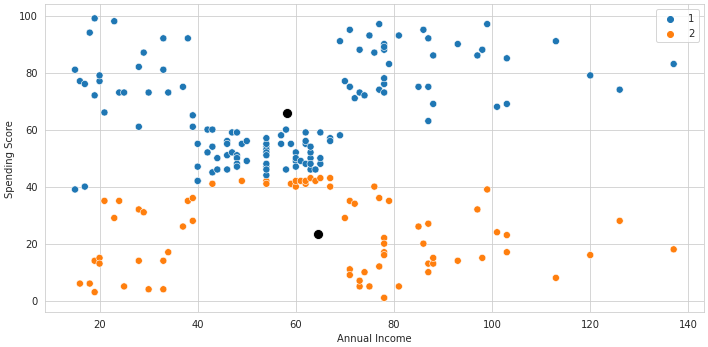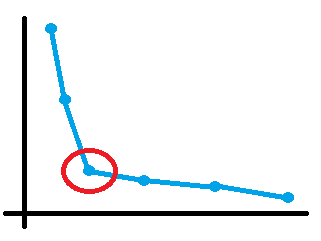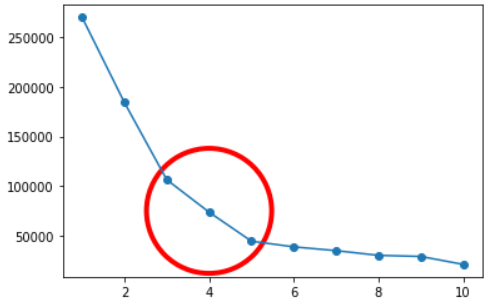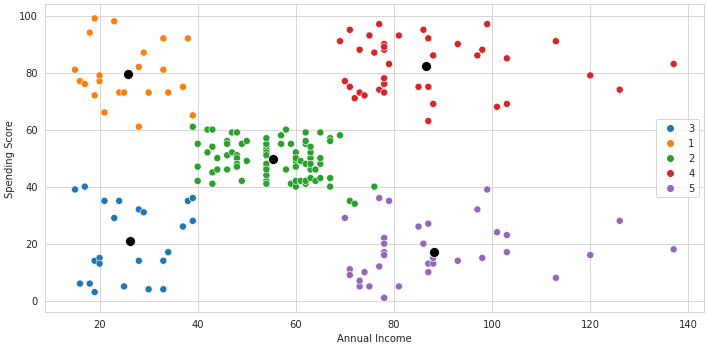# Tutorial: Clustering in Machine LearningShare :Originally posted on dataquest

Clustering is one of the most common tasks in unsupervised machine learning. We use clustering algorithms to segment a dataset into groups based on the patterns in the data.

For instance, let’s say we have data about thousands of our company’s customers. We could use a clustering algorithm to split these customers into groups in order to apply different marketing strategies to each group.

To perform such segmentation, there are two main machine learning algorithms that we could use:

• The k-means algorithm
• Hierarchical clustering

In this tutorial, we’ll focus on the k-means algorithm.

You don’t need advanced knowledge to follow along with this tutorial, but we do expect you to be familiar with basic Python and the pandas library.

## The K-means Algorithm

The k-means algorithm is an iterative algorithm designed to find a split for a dataset given a number of clusters set by the user. The number of clusters is called K.

The algorithm starts by randomly choosing K points to be the initial centers of the clusters. These points are called the clusters’ centroids. The user sets K.

Then, as an interactive algorithm, k-means repeats the same process until it reaches a determined stopping condition. In this process, the following steps are repeated:

1. Calculate the distance from each data point to each centroid.
2. Assign the data points to the closest centroid.
3. Recalculate the centroids as the mean of the points in their cluster.

The stopping condition is met when the centroids are in the mean of their clusters, which means that they will no longer change.

The animation below illustrates this process:## Implementing K-means from Scratch

Now that we understand k-means, we’ll start building an implementation from scratch.

To put our algorithm to work, we’ll use a dataset of customers that we intend to segment based on their annual income and spending score. You can access the dataset here. It contains the following variables:

• `CustomerID`: a unique identifier for each customer

`Annual Income`: the customer’s annual income in thousands of dollars

`Spending Score`: a score based on customer shopping patterns. Goes from 1 to 100

Here are the frst five rows of the dataset:

``````import pandas as pd
import numpy as np

CustomerID Annual Income Spending Score
0 1 15 39
1 2 15 81
2 3 16 6
3 4 16 77
4 5 17 40

In order to segment this dataset into two clusters, we’ll write a series of functions that, once combined, will behave as a simplified version of k-means.

The first function is the `get_centroids()` function. This is a very simple piece of code responsible for randomly choosing K customers as the initial centroids. It returns the selected rows and the values of the two variables we’re using as a list of lists. We’ll now refer to these variables as coordinates.

Here’s the function:

``````def get_centroids(df, k):
np.random.seed(1)
centroids = df.sample(k).reset_index(drop=True)

return centroids, centroids.values.tolist()``````

And here’s the output when we set `k=2`:

``````centroids, coords = get_centroids(customers[['Annual Income', 'Spending Score']], k=2)
print(centroids)
print(coords)``````
``````Annual Income  Spending Score
0             46              51
1             38              35
[[46, 51], [38, 35]]``````

Next, we’ll write a function to calculate the distance between every customer to each of the two initial centroids. The `calculate_distance()` function takes in the data and the coordinates of the centroids:

``````def calculate_distance(df, centroids_coords):
names = []
for i, centroid in enumerate(centroids_coords):
name = f'dist_centroid_{i + 1}'
df.loc[:, name] = np.sqrt((df.iloc[:,0] - centroid)**2 + (df.iloc[:,1] - centroid)**2)
names.append(name)

return df, names

df, dist_columns = calculate_distance(customers[['Annual Income', 'Spending Score']], coords)
print(df)
print(dist_columns)``````
``````Annual Income  Spending Score  dist_centroid_1  dist_centroid_2
0               15              39        33.241540        23.345235
1               15              81        43.139309        51.429563
2               16               6        54.083269        36.400549
3               16              77        39.698866        47.413078
4               17              40        31.016125        21.587033
..             ...             ...              ...              ...
195            120              79        79.120162        93.059121
196            126              28        83.240615        88.277970
197            126              74        83.240615        96.254870
198            137              18        96.798760       100.448992
199            137              83        96.462428       110.022725

[200 rows x 4 columns]
['dist_centroid_1', 'dist_centroid_2']``````

Notice that the function adds new columns to the DataFrame representing the distance of that customer to each cluster. It then returns the modified DataFrame and a list with the name of the new columns.

To calculate the distance, we used the Euclidean Distance formula.

With these two functions working, we’ll start to write our main function. The `kmeans()` function takes in two arguments: the DataFrame to be segmented and a value for `k`.

The first two steps are to create an array with original variables in the dataset and then call the `get_centroids()` function to initialize the centroids. Here’s how it looks at this point:

``````def kmeans(df, k):
variables = df.columns
centroids, coords = get_centroids(df, k)``````

Notice that we are supposed to pass the DataFrame containing only the variables used in the clustering process and not the `Customer ID`, for instance, as we’ve been doing already.

Building on that, inside a loop, we’ll perform three other steps:

1. Create a copy of the coordinates we got from the `get_centroids()` function.
2. Use the coordinates to calculate the distances from each customer to each centroid with the `calculate_distance()` function.
3. Create a `cluster` column in the DataFrame containing the number of their closest column to each customer.

The code below shows the function after these modifications. We added a `print` and a `break` statement so we can visualize how the data looks after the first iteration.

``````def kmeans(df, k):
variables = df.columns
centroids, coords = get_centroids(df, k)

while True:
# Creating a copy of the coordinates to compare with the new coordinates that we'll generate
last_coords = coords.copy()

df, dist_columns = calculate_distance(df, coords)

df['cluster'] = df[dist_columns].idxmin(axis=1).str.split('_').str[-1]
print(df)
break``````

We use the `idxmin()` method to get the lower value in each row of a DataFrame containing only `dist_columns`, which are the columns we created to represent the distance from each customer to each centroid.

When we run the function as it is, we can see that we have a cluster, 1 or 2, assigned to each customer:

``kmeans(customers[['Annual Income', 'Spending Score']], k=2)``
``````Annual Income  Spending Score  dist_centroid_1  dist_centroid_2 cluster
0               15              39        33.241540        23.345235       2
1               15              81        43.139309        51.429563       1
2               16               6        54.083269        36.400549       2
3               16              77        39.698866        47.413078       1
4               17              40        31.016125        21.587033       2
..             ...             ...              ...              ...     ...
195            120              79        79.120162        93.059121       1
196            126              28        83.240615        88.277970       1
197            126              74        83.240615        96.254870       1
198            137              18        96.798760       100.448992       1
199            137              83        96.462428       110.022725       1

[200 rows x 5 columns]``````

We have four new steps left to finish the main function:

1. Recalculate the centroids as the mean of the cluster. For that, we use `groupby()` and the list of variables we create at the beginning of the function.
2. Transform the coordinates of these new centroids to a list of lists just like the one we get from the `get_centroids()` function.
3. Check if these new coordinates are equal to the last one. If so, this means the centroids are located at the mean of their cluster, and we’ve reached our stoppage points. We then break the loop and return only the `cluster` column and the `centroids` DataFrame.
4. We also added a counter so we can know how many iterations were necessary to find a split in the dataset.
5. Finally, we added the `max_iterations` parameter to stop the loop if it takes too long for the centroid to converge to the mean of their clusters.

Here’s the completed function:

``````def kmeans(df, k, max_iterations=50):
variables = df.columns
centroids, coords = get_centroids(df, k)

counter = 0
while True:
last_coords = coords.copy()

df, dist_columns = calculate_distance(df, coords)

df['cluster'] = df[dist_columns].idxmin(axis=1).str.split('_').str[-1]

centroids = round(df.groupby('cluster')[variables].mean(), 2)
coords = centroids.values.tolist()

if last_coords == coords or counter + 1 == max_iterations:
print(f'Number of iterations: {counter + 1}')
return df['cluster'], centroids
else:
counter += 1``````

We then call the function and assign the result to a new column called `cluster` in our original DataFrame:

``````clusters, centroids = kmeans(customers[['Annual Income', 'Spending Score']], k=2)
customers['cluster'] = clusters
print(customers)``````
``````Number of iterations: 8
CustomerID  Annual Income  Spending Score cluster
0             1             15              39       1
1             2             15              81       1
2             3             16               6       2
3             4             16              77       1
4             5             17              40       1
..          ...            ...             ...     ...
195         196            120              79       1
196         197            126              28       2
197         198            126              74       1
198         199            137              18       2
199         200            137              83       1

[200 rows x 4 columns]``````

We now use `matplotlib` and `seaborn` to visualize how the customers were split and where the final centroids (the black points) are located:

``````import matplotlib.pyplot as plt
import seaborn as sns

sns.set_style('whitegrid')

fig, ax = plt.subplots(figsize=(10, 5))
sns.scatterplot(x='Annual Income', y='Spending Score', hue='cluster', palette='tab10', data=customers, s=50, ax=ax)
sns.scatterplot(x='Annual Income', y='Spending Score', color='black', data=centroids, s=100, ax=ax)

plt.tight_layout()
plt.show()``````## Inertia and the Elbow Curve

This looks like a fair split, right? Customers with higher spending scores are in one cluster, and the ones with low spending scores are in another. But is there a way to know if there would be a better split than this one? The answer is yes.

First, we need to understand an important metric for k-means: inertia. Inertia is the measure of how far the data points assigned to a cluster are from that cluster’s centroid.

Mathematically speaking, inertia is the sum of the squared distances from each of the data points to its centroid. The lower the inertia, the closer the points are to their centroids, which is what we’re looking for.

Our dataset contains 200 customers. If we split it into 200 customers, the inertia would be zero, and each customer would be its own cluster, which doesn’t make sense at all. But have we not said the lower the inertia, the better? Yes, but only to a certain extent.

As we’ve seen, the higher the number of clusters, the lower the inertia, and we need to decide when the inertia is low enough so we can stop adding clusters. This is when the Elbow Curve comes in.

The Elbow Curve is nothing more than a line plot of the inertias against the number of clusters. Since inertia decreases while the number of clusters increases, the curve looks like this:As we start from one cluster and add the second and third, inertia will drop very fast. At some point, however, it plateaus, creating a “sharp elbow” in the curve. At this point, adding more clusters will not reduce significantly the inertia, and this is where we should stop.

Here’s an example of a sharp elbow:To create such a plot, we need to run the k-means for multiple numbers of K and then calculate the inertia for each one. The following code performs this calculation using K from 1 to 9 and stores the data into a DataFrame:

``````inertias = []
for k in range(1, 11):
# Creating a temporary DataFrame to calculate the inertia for each k
df_temp = customers[['Annual Income', 'Spending Score']].copy()
# Calculating the clusters for each k
clusters, centroids = kmeans(customers[['Annual Income', 'Spending Score']], k=k)
# Adding the clusters and the coordinates of centroids to the temporary DataFrame
df_temp['cluster'] = clusters
df_temp = df_temp.merge(centroids.rename(columns={'Annual Income': 'Annual Income_centroid',
'Spending Score': 'Spending Score_centroid'}), on='cluster')
# Using euclidean distance an calcualting the squared distance of each point to its centroid
df_temp['sqr_distance'] = (np.sqrt((df_temp['Annual Income'] - df_temp['Annual Income_centroid'])**2 + (df_temp['Spending Score'] - df_temp['Spending Score_centroid'])**2))**2
# Storing the sum od the squared distances
inertias.append([k, df_temp['sqr_distance'].sum()])

df_inertia = pd.DataFrame(inertias, columns=['k', 'inertia'])``````
``````Number of iterations: 2
Number of iterations: 8
Number of iterations: 10
Number of iterations: 8
Number of iterations: 10
Number of iterations: 10
Number of iterations: 7
Number of iterations: 13
Number of iterations: 9
Number of iterations: 50``````

If we use the inertias to create the elbow curve, we would have this plot:Notice that, this time, we don’t have an obvious point to choose from and that the “elbow” is not that sharp. This is quite normal, and sometime we must investigate where the decrease in inertia really drops and look for business insights to determine `K`.

In our situation, we’ll go with five. Here’s what this new split looks like:

``````# Reading the data again

# Creating the clusters using k=5
clusters, centroids = kmeans(customers[['Annual Income', 'Spending Score']], k=5)
customers['cluster'] = clusters

# Plotting the results
fig, ax = plt.subplots(figsize=(10, 5))
sns.scatterplot(x='Annual Income', y='Spending Score', hue='cluster', palette='tab10', data=customers, s=50, ax=ax)
sns.scatterplot(x='Annual Income', y='Spending Score', color='black', data=centroids, s=100, ax=ax)

plt.tight_layout()
plt.show()``````
``Number of iterations: 10``In fact, it’s much better, as we can clearly see five groups with different characteristics regarding both variables.

## Conclusion

In this article, in order to take a closer look at how the clustering algorithm k-means works, we did the following:

• Explored some important clustering concepts
• Implemented a simplified-but-functional version of k-means from scratch
• Learned how to use the Elbow Curve

Source: dataquest

Share :